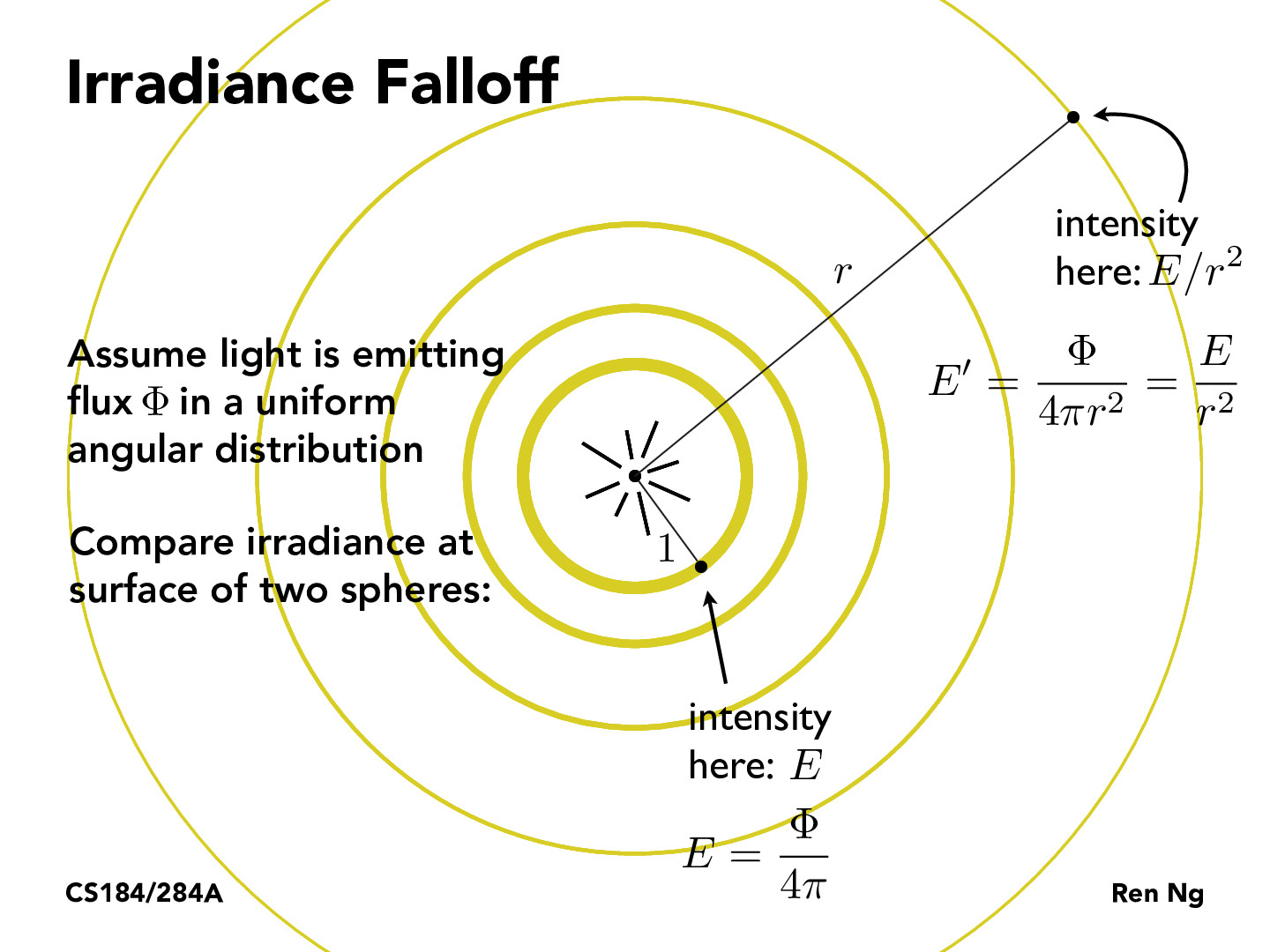Lecture 11: Radiometry and Photometry (29)sungpark98

The irradiance falloff happens at this exact ratio of r^2 because irradiance is the power per unit area incident on a surface point. The 4 pi r^2 is looking at the area of the circle around. Is it 4pi instead of 2pi because we're using solid angles instead of a regular angle?visatish

I don't think this is related to solid angles. I believe it is $4\pi$ because the equation for the surface area of a sphere is $4\pi r^2$. For the inner irradiance, $r=1$ and thus it is just $4\pi$.andrewdotwang

Why can we use intensity to describe irradiance here?gabby-delforge

@andrewdotwang:

It can get a bit confusing when we mix colloquial lighting language with these terms, but in this case, "intensity" is just a way of expressing how bright the light will be at different distances.

You must be enrolled in the course to comment